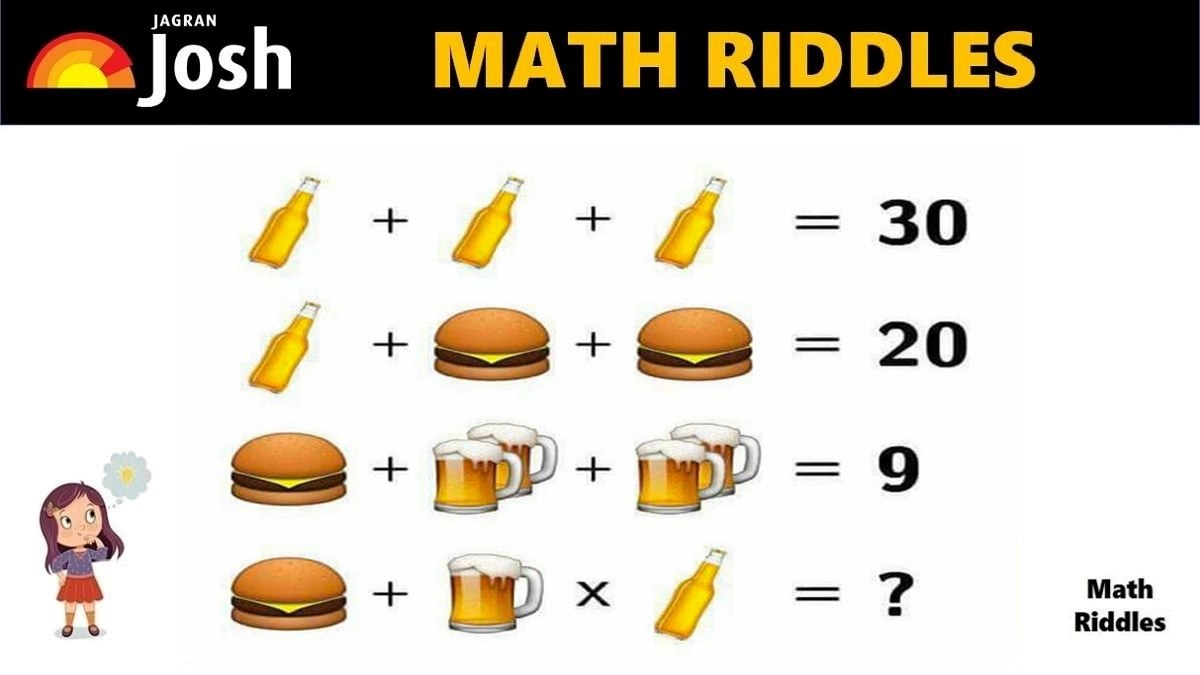# Math Riddles With Answers: Can You Find The Value of Burger and Beer in 20 Seconds?

In this Math Puzzle, we must find the values of Burger and Beer each to solve this tricky math riddle. Are you a math genius? Only 1% with a high IQ can solve this tricky Burger Beer math puzzle in 20 seconds.Can you find the values of Burger and Beer each to solve this hard math riddle. Are you a math genius? Only 1% with a high IQ can solve this tricky Burger Beer math puzzle in 20 seconds.

In this Math Puzzle, we must find the values of Burger and Beer each to solve this tricky math riddle. Are you a math genius? Only 1% with a high IQ can solve this tricky math puzzle in 20 seconds.

Share this math puzzle with your friend and family. These math riddles are a great source for solving math problems in a fun way. Try to test your mathematical knowledge and genius level with this math puzzle. Let us solve this math picture puzzle within 20 seconds.

If you love solving cryptic math puzzles, give this math puzzle a try! You have 20 seconds to find the correct answer.

## Math Riddles with Answers: Solution

Explanation:

Row 1: We have 1 Beer Bottle + 1 Beer Bottle + 1 Beer Bottle = 30

So, we get 3 Beer Bottle = 30. If we divide RHS by 3, we get the result that the value of 1 Beer Bottle is 10. See the solved equations below:

=> 1 Beer Bottle + 1 Beer Bottle + 1 Beer Bottle = 30

=> 3 Beer Bottle = 30

=> 1 Beer Bottle = 30/3

=> 1 Beer Bottle = 10

Now, we will use the value of 1 Beer Bottle to solve the next row of the problem.

Math Riddles With Answers: Solve This Donald Duck, Jerry, Mickey Mouse Math Puzzle in 20 Seconds

Row 2: We have 1 Beer Bottle + 1 Burger + 1 Burger = 20

We found that the value of 1 Beer Bottle is 10 in Row 1. We shall apply the value in Row 2 and solve to find the value of 1 Burger. See the solved equation below:

=> 1 Beer Bottle + 1 Burger + 1 Burger = 20

=> 10 + 2 Burger = 20

=> 2 Burger = 20 – 10

=> 2 Burger = 10

=> 1 Burger = 5

Now, we will use the value of 1 Burger to solve the next row of the problem.

Math Riddles With Answers: Can You Find The Value of Burger, Fries, Coke in 20 Seconds?

Row 3: We have 1 Burger + 2 Beer Glass + 2 Beer Glass = 9

We have found the value of 1 Burger is 5 in Row 2. We shall apply the value in Row 3 and solve to find the value of 1 Beer Glass. See the solved equation below:

=> 1 Burger + 2 Beer Glass + 2 Beer Glass = 9

=> 5 + 4 Beer Glass = 9

=> 4 Beer Glass = 9 – 5

=> 4 Beer Glass = 4

=> 1 Beer Glass = 4/4

=> 1 Beer Glass = 1

Now, we will use the value of Beer Bottle, Burger, and Beer Glass to solve the final row of the problem.

Picture Puzzles Can You Find The Hidden Dice Among The Cheese and Mice In 11 Seconds?

Row 4: We have 1 Burger + 1 Beer Glass x 1 Beer Bottle = ?

=> 5 + 1 x 10 = ?

Apply BODMAS

=> 5 + 10 = ?

=> 15

Hence, the final answer for this math puzzle is 15

Great Work!

## Tell us in comments: Did you solve this math puzzle correctly in 20 seconds?

Check out more amazing tricky math puzzles!

Math Riddles With Answers: Can You Find The Value Of Lemon In 20 Seconds?

Test Your Observation Skills! Find These Hidden Objects In This Picture Puzzle In 11 Seconds?

Can You Solve This Tricky Flower Math Riddle In 20 Seconds?

Genius Alert! Can You Find The Hidden Fish In This Picture Puzzle In 11 Seconds?

Math Riddles With Answers: Can You Find The Value of Each Candy in 20 Seconds?

Get the latest General Knowledge and Current Affairs from all over India and world for all competitive exams.
खेलें हर किस्म के रोमांच से भरपूर गेम्स सिर्फ़ जागरण प्ले पर